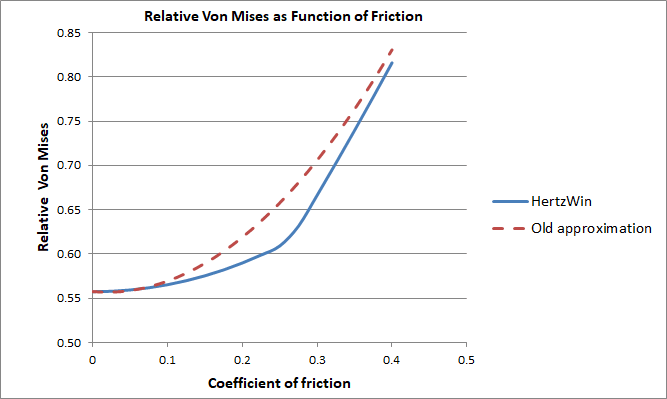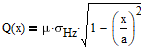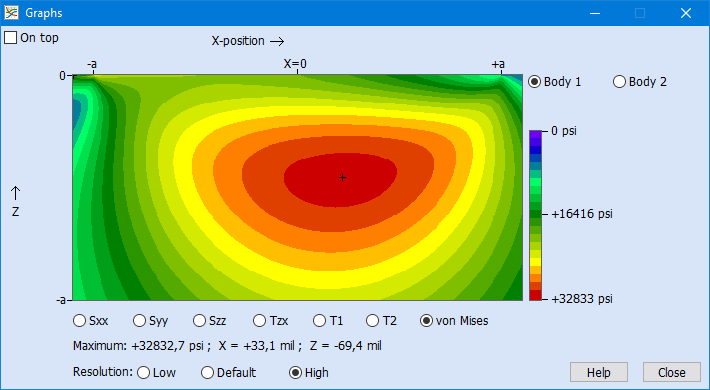Select Page

With the program HertzWin you can calculate Hertz point and line contacts. The influence of a tangential force can also be calculated. HertzWin has been available online for free since March 2009.

## No approximation but the exact solution

In older releases of HertzWin (prior to release 3.1.2) a general approximation formula was used. From release 3.2.0 (December 2020), the situation with gross slip between both bodies can be calculated in detail in HertzWin. This can be done for a circular point contact and for a line contact. You can find an overview of the formulas used in the program’s help function.

In the figure below, the old approximation in HertzWin is plotted against the coefficient of friction, together with the new, exact method in HertzWin. The vertical axis shows the Von Mises (vM) stress in relation to the maximum compressive stress (σHz): vM/σHz. The clearly visible sudden change in the slope of the blue line is the point where the maximum Von Mises stress shifts to the surface.## Three Situations: no Slip, Partial Slip or Full Slip

The calculation of a (Hertz) point or line contact that is loaded with a tangential load is usually divided into three different situations:

• there is no slip between the two bodies (stick);
• there is partial slip between the two bodies;
• both bodies slide with respect to each other, gross slip.

When applying a tangential load, a coupling (dependency) with the normal load is created. The calculation is therefore based on the following:

• the normal force is applied first. A small contact area is created which is calculated using the usual Hertz theory;
• then the tangential force is slowly added, slowly increasing from 0 Newton;
• at low values of the tangential force (low in relation to the maximum friction force), there is almost no slip;
• at higher tangential forces, a slip zone is created on the edge of the contact;
• if the tangential force exceeds the maximum friction, the contact turns into gross slip.

With a normal Hertzian contact, the pressure distribution on the contact surface is quadratic:With: a = half contact width, x = distance to the center of the contact and σHz = maximum compressive stress in the center of the contact.

Assuming that the (Amonton) law of friction holds at any point on the contact surface, the local tangential force Q(x) (stress) at the surface is given by the following function.With μ the coefficient of friction. This distribution is used in calculating the effect of gross slip. The next figure shows how the actual force distribution looks like when a tangential force is present.

## Elliptical Contact Calculation

Calculations with a tangential force on an elliptical contact are currently not (yet) possible in HertzWin. However, it appears that the differences between a circular contact and a line contact are not very great, see ‘Rough Contact Between Elastically and Geometrically Identical Curved Bodies‘ by Bryant and Keer. If the contact is highly elliptical, the results of a line contact with the same Hertzian compressive stress can be used. When the contact is close to circular, the results for a circular contact area can be used. Two extreme examples, both with: Young’s modulus of 200 GPa, Poisson ratio 0.3 and a coefficient of friction of 0.2:

1. Line contact, radii 60 mm and 20 mm, contact length 100 mm, normal force 5000 N. This situation results in a Hertzian compressive stress of 341.5 MPa and a maximum Von Mises stress of 201.5 MPa. The maximum tensile stress is 136.6 MPa;
2. Circular contact, sphere on a flat surface with sphere radius 60 mm and a tuned normal force of 61.34 N so that it also results in a Hertzian compressive stress of 341.5 MPa. The maximum Von Mises stress is now 217.7 MPa. The maximum tensile stress is 160.9 MPa.

Between this line contact and the point contact there is a difference of less than 10% for the Von Mises and less than 20% for the tensile stress. Entirely in accordance with the conclusions in Bryant and Keer‘s article. The stresses for an elliptical contact will be in between these results. The direction of the tangential load in an elliptical contact is parallel to the minor (minor) axis ‘b’. If the tangential force is parallel to the major axis ‘a’, errors in estimation can be large.

## Contour Plot with Colors

The material stresses below the contact surface are calculated in HertzWin and the main stresses (shear stress, Von Mises and tensile stress) are displayed in the main window. For more insight into the material stresses you can create contour plots via the graphs button. Below is an example of such a contour plot for a circular point contact with a Poisson ratio of 0.3 and a friction coefficient of 0.2.## Von Mises Stress Shifts to the Surface with High Friction and/or Low Poisson Ratio

As the tangential load increases, the location where the maximum Von Mises stress occurs, slowly shifts towards the surface. The video below shows the progress with a sliding cilinder (line contact) and increasing coefficient of friction.

Important in this example is the Poisson ratio of 0.3. The moment of the shift towards the contact surface strongly depends of the Poisson ratio. With a value lower than ~0.2, the maximum Von Mises is always on the contact surface.

As can be seen, the Von Mises stress slowly increases in the beginning: only +1.4% with a friction coefficient of 0.1 and +5.8% with a friction coefficient of 0.2. The closer the Von Mises stress is to the surface, the higher the rate of increase. At a friction coefficient of 0.4, the increase is +46.3%.